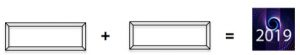How many addition sums can you make by putting a whole number in each box to give the answer 2019?

You could put 1009 in one box and 1000 in the other box or you could put 2018 in one box and 1 in the other box and there are lots more ways to make 2019.

So how many ways can you add two whole numbers to get the answer 2019?

Can you add two even numbers to get the answer 2019?

Can you add two odd numbers to get the answer 2019?

If you find this difficult try the same problem for a smaller total. How many ways can you add two whole numbers to make 21?  Can you add two even numbers to make 21?

Tagged with: